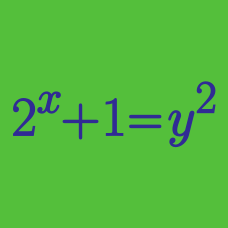Algebra

# Advanced Simplification Tricks

If $\frac{1}{a}-\frac{1}{b}=-37$, the value of $\frac{a+8ab-b}{a-3ab-b}$ can be expressed as $\frac{n}{m}$, where $m$ and $n$ are coprime positive integers. What is the value of $m+n$?

If the polynomial $x^2-y^2+11x+7y+18$ can be factorized as $(x+y+a)(x-y+b),$ what is the value of $a+b?$

If $-x^3+6x^2-6x+36$ is factorized as $(a-x)(x^2+b),$ what is the value of $a+b$?

If $x+2y-6+3(1-x-2y)=-51$, what is $x+2y$?

If $x+2y=-13$, what is the value of $x^2+4xy-2x-4y-3+4y^2?$

×

Problem Loading...

Note Loading...

Set Loading...